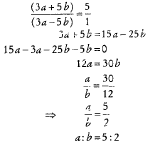# Mathematical Aptitude - Ratio Proportion, Percentage and Discounting

>>>>>>>>Ratio Proportion, Percentage and Discounting

• A

1 : 1• B

1 : 2• C

1 : 3• D

1 : 4• Option : D
• Explanation : (D) If the ratio is 1 : 4, Then the number of defective bulbs = x and number of good bulbs = 4x
∴ x + 4x = 12
⇒ 5x = 12
which is not possible for any integral value of x.
So, 1 : 4 is not possible.

• A

3 : 2• B

5 : 3• C

2 : 1• D

5 : 2• Option : D
• Explanation : (D)• A

4 : 3 : 5• B

6 : 5 : 9• C

10 : 9 :12• D

12 : 10 : 15• Option : D
• Explanation : (D) Let the production of Y =100
Then, production of X =120
Production of Z =150
Required ratio of X, Y and X
=X : Y : Z
=120 : 100 : 150
=12 : 10 : 15

• A

20• B

25• C

20.50• D

None of the above• Option : A
• Explanation : Lets consider x% of 180.5 = 36.1
x% = 36.1/180.5
x = 36.1/180.5 × 100 = 20%

• A

25• B

30%• C

20%• D

15%• Option : C
• Explanation : Let original price = 100
Original quantity = 100
Total expenditure = 100 × 100
New price = 100 + 25 = 125
New quantity = Q
As expenditure is to be kept same, 100 × 100 = 125 × Q
Q = (100 × 100)/125 = 80. % reduction in quantity = 100 - 80 = 20

## Description

Who can benefit –

• Any undergraduate or postgraduate student who is seeking Ratio Proportion objective type questions answers can use this section.
• Ratio Proportion Questions Answers can be used for the preparation of UGC NET Paper1 Exams.
• Percentage and  Discounting Aptitude Questions are also used by students in the preparation of their Exams.
• All the teachers who are appearing for Kendriya Vidyalya Sangathan Entrance Exam PGT, TGT, can also use Ratio Proportion Multiple Choice Questions Answers.
• Profit and Loss Aptitude Questions can also be used by the students who wants to pursue pursuing B.Ed or M. Ed.
• Any student who wants to prepare for UGC NET Paper1 can also use this Profit and Loss Aptitude Questions .
• UGC NET first paper students can also prepare for their exams using Ratio Proportion Objective Type Questions Answers.
• You can get free access to Percentage and  Discounting Aptitude Questions   pdf.
• Any student who wants to prepare for UGC NET Paper1, MBA Entrance Exam, CAT, MAT, SAT, Ph. D Entrance Exam, PET, NDA, CDS or any other competitive Exam can also use this Profit and Loss Aptitude Questions .

Various Search Terms used for this section are